##### chapter 1

0
Set Details Share
created 8 years ago by Jesse123
602 views
Page to share:
Embed this setcancel
COPY
code changes based on your size selection
Size:
X
1

undefined term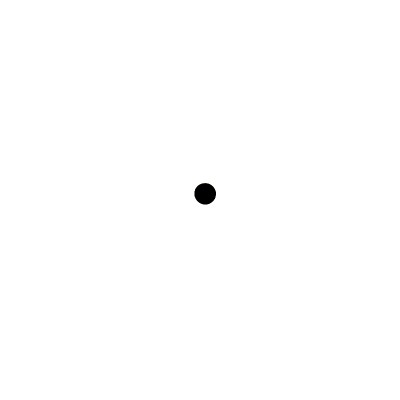ex; point, line, plane

2

pointa location, has neither size or shape

3

line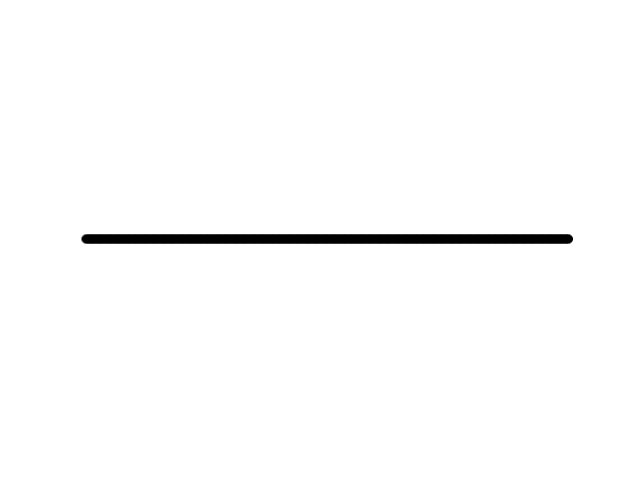made up of points and has no thickness or width

4

planea flat surface made up of points that extends indefinitely in all directions

5

collinearpoints that lie on the same line

6

coplanarpoints that lie on the same plane

7

intersectionThe set of points they have in common, two lines intersect at one point

8

definition

explained using undefined terms and/or other defined terms

9

defined term

explained using undefined terms and/or other defined terms

10

spacedefined as boundless

11

line segmenta straight line which links two points without extending beyond them

12

betweenness of pointsa point is between two other points on the same line

13

betweenin a space separating two objects

14

congruent segments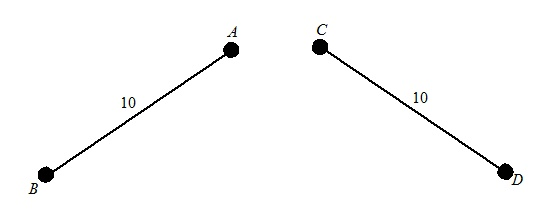segments that have the same measure

15

construction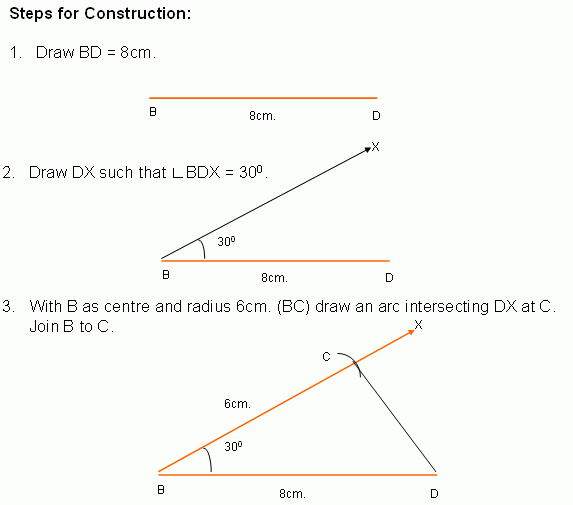methods of creating geometric figures without the benefit of measuring tools

16

distancethe length of the segment with those points as its endpoints

17

midpointthe point halfway between the endpoints of the segment

18

segment bisectorany segment, line or plane that intersects a segment at its midpoint

19

ray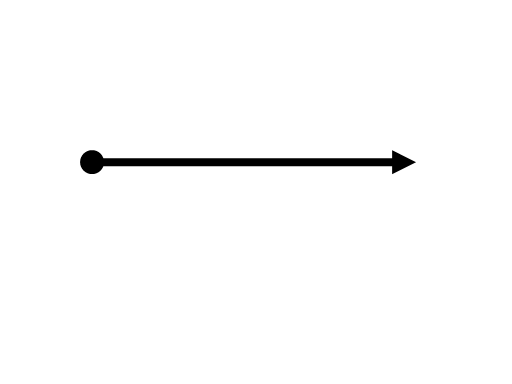a part of a line

20

opposite raystwo rays with a common endpoint that point in opposite directions and form a straight line.

21

angle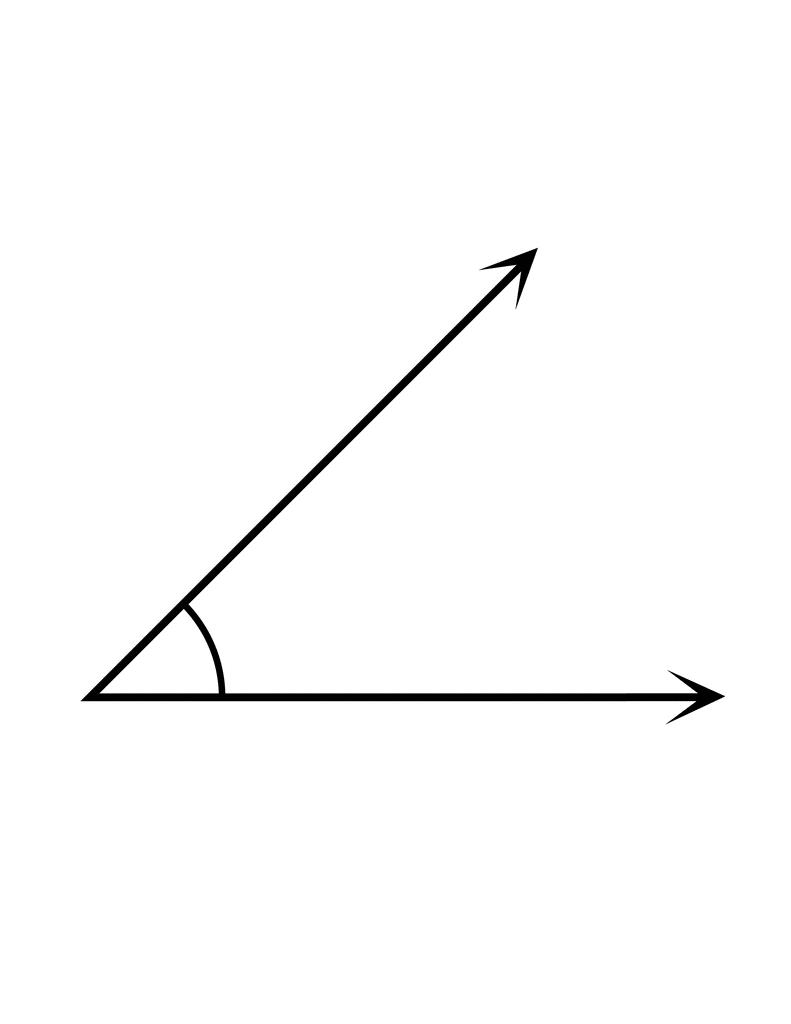formed by two noncollinear rays that have a common endpoint

22

sidethe rays

23

vertexthe common endpoint

24

interiorthe space inside of an angle

25

exteriorthe space outside of an angle

26

degreea measurement of plane angle; 1/360

27

right anglean angle whose measure is exactly 90°

28

acute anglean angle whose measure is less than 90°

29

obtuse angle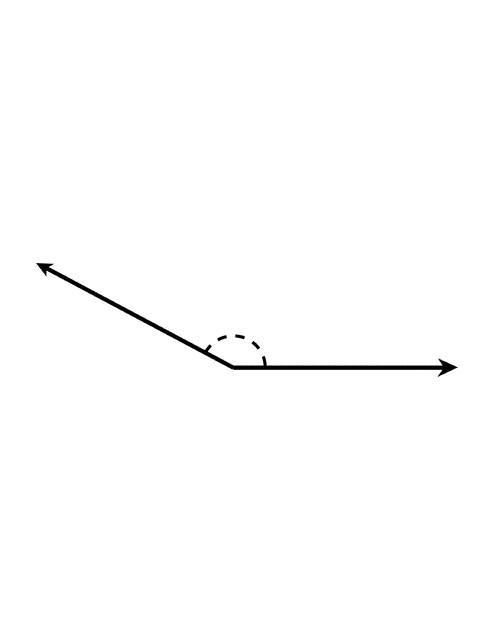an angle whose measure is greater than 90° and less than 180°

30

angle bisectora ray that divides an angle into two congruent angles

31two angles that lie in the same plane and have a common vertex and a common side, but no common interior points

32

linear paira pair of adjacent angles with no common sides that are opposite rays

33

vertical anglestwo adjacent angles formed by two intersecting lines

34

complementary angles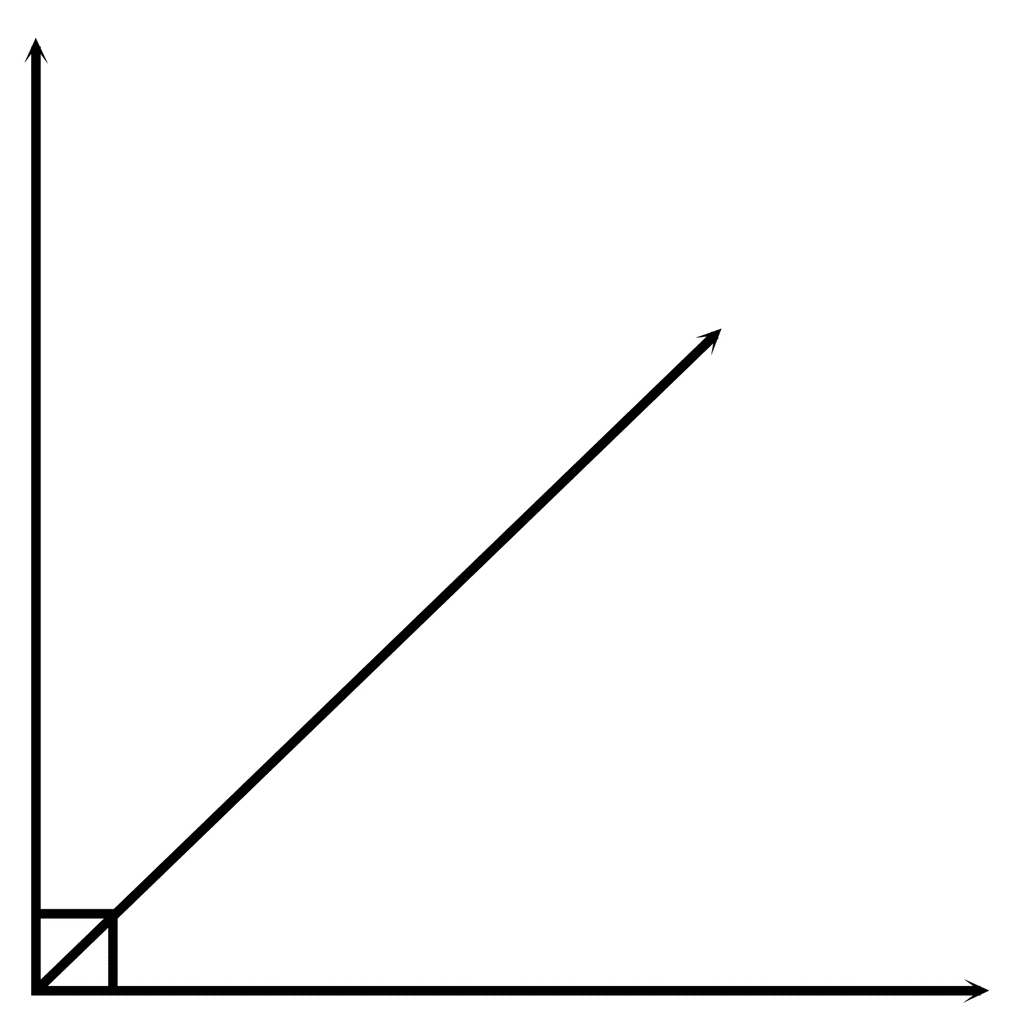two angles with measures that have a sum of 90

35

supplementary angles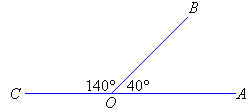two angles with measures that have a sum of 180

36

perpendicularlines, segments, rays that form right angles